# IF AND formula in Excel

The tutorial shows how to use IF together with the AND function in Excel to check multiple conditions in one formula.

Some things in the world are finite. Others are infinite, and the IF function seems to be one of such things. On our blog, we already have a handful of Excel IF tutorials and still discover new uses every day. Today, we are going to look at how you can use IF together with the AND function to evaluate two or more conditions at the same time.

## IF AND statement in Excel

In order to build the IF AND statement, you obviously need to combine the IF and AND functions in one formula. Here's how:

IF(AND(condition1, condition2,…), value_if_true, value_if_false)

Translated into plain English, the formula reads as follows: IF condition 1 is true AND condition 2 is true, do one thing, otherwise do something else.

As an example, let's make a formula that checks if B2 is "delivered" and C2 is not empty, and depending on the results, does one of the following:

• If both conditions are TRUE, mark the order as "Closed".
• If either condition is FALSE or both are FALSE, then return an empty string ("").

`=IF(AND(B2="delivered", C2<>""), "Closed", "")`

The screenshot below shows the IF AND function in Excel: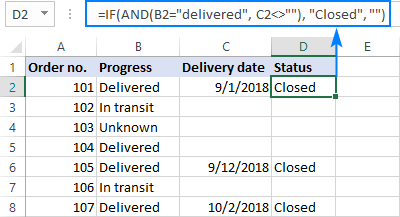If you'd like to return some value in case the logical test evaluates to FALSE, supply that value in the value_if_false argument. For example:

`=IF(AND(B2="delivered", C2<>""), "Closed", "Open")`

The modified formula outputs "Closed" if column B is "delivered" and C has any date in it (non-blank). In all other cases, it returns "Open":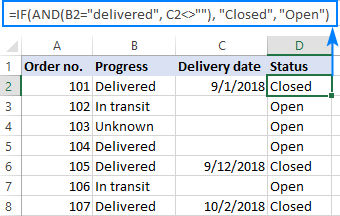Note. When using an IF AND formula in Excel to evaluate text conditions, please keep in mind that lowercase and uppercase are treated as the same character. If you are looking for a case-sensitive IF AND formula, wrap one or more arguments of AND into the EXACT function as it is done in the linked example.

Now that you know the syntax of the Excel IF AND statement, let me show you what kind of tasks it can solve.

## Excel IF: greater than AND less than

In the previous example, we were testing two conditions in two different cells. But sometimes you may need to run two or more tests on the same cell. A typical example is checking if a cell value is between two numbers. The Excel IF AND function can easily do that too!

Let's say you have some sales numbers in column B and you are requested to flag the amounts greater than \$50 but less than \$100. To have it done, insert this formula in C2 and then copy it down the column:

`=IF(AND(B2>50, B2<100), "x", "")`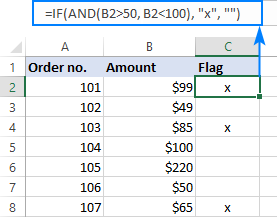If you need to include the boundary values (50 and 100), use the less than or equal to operator (<=) and greater than or equal to (>=) operator:

`=IF(AND(B2>=50, B2<=100), "x", "")`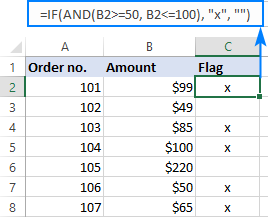To process some other boundary values without changing the formula, enter the minimum and maximum numbers in two separate cells and refer to those cells in your formula. For the formula to work correctly in all the rows, be sure to use absolute references for the boundary cells (\$F\$1 and \$F\$2 in our case):

`=IF(AND(B2>=\$F\$1, B2<=\$F\$2), "x", "")`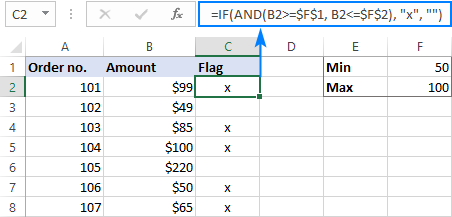By using a similar formula, you can check if a date falls within a specified range.

For example, let's flag dates between 10-Sep-2018 and 30-Sep-2018, inclusive. A small hurdle is that dates cannot be supplied to the logical tests directly. For Excel to understand the dates, they should be enclosed in the DATEVALUE function, like this:

`=IF(AND(B2>=DATEVALUE("9/10/2018"), B2<=DATEVALUE("9/30/2018")), "x", "")`

Or simply input the From and To dates in two cells (\$F\$1 and \$F\$2 in this example) and "pull" them from those cells by using the already familiar IF AND formula:

`=IF(AND(B2>=\$F\$1, B2<=\$F\$2), "x", "")`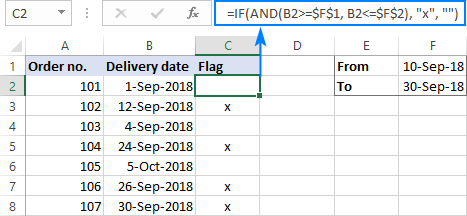## IF this AND that, then calculate something

Apart from returning predefined values, the Excel IF AND function can also perform different calculations depending on whether the specified conditions are TRUE or FALSE.

To demonstrate the approach, we will be calculating a bonus of 5% for "Closed" sales with the amount greater than or equal to \$100.

Assuming the amount is in column B and the order status in column C, the formula goes as follows:

`=IF(AND(B2>=100, C2="closed"), B2*10%, 0)`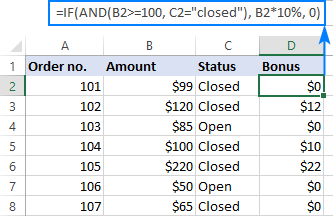The above formula assigns zero to the rest of the orders (value_if_false = 0). If you are willing to give a small stimulating bonus, say 3%, to orders that do not meet the conditions, include the corresponding equation in the value_if_false argument:

`=IF(AND(B2>=100, C2="closed"), B2*10%, B2*3%)`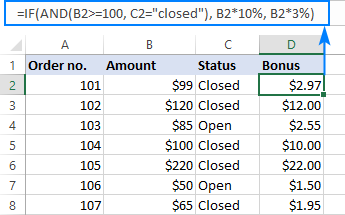## Multiple IF AND statements in Excel

As you may have noticed, we have evaluated only two criteria in all the above examples. But there is nothing that would prevent you from including three and more tests in your IF AND formulas as long as they comply with these general limitations of Excel:

• In Excel 2019 - 2007, up to 255 arguments can be used in a formula, with a total formula length not exceeding 8,192 characters.
• In Excel 2003 and lower, no more than 30 arguments are allowed, with a total length not exceeding 1,024 characters.

As an example of multiple AND conditions, please consider these ones:

• Amount (B2) should be greater than or equal to \$100
• Order status (C2) is "Closed"
• Delivery date (D2) is within the current month

Now, we need an IF AND statement to identify the orders for which all 3 conditions are TRUE. And here it is:

`=IF(AND(B2>=100, C2="Closed", MONTH(D2)=MONTH(TODAY())), "x", "")`

Given that the 'current month' at the moment of writing was October, the formula delivers the below results: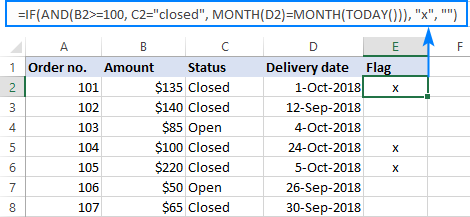## Nested IF AND statements

When working with large worksheets, chances are that you may be required to check a few sets of different AND criteria at a time. For this, you take a classic Excel nested IF formula and extend its logical tests with AND statements, like this:

IF(AND(…), output1, IF(AND(…), output2, IF(AND(…), output3, output4)))

To get the general idea, please look at the following example.

Supposing you want to rate your service based on the shipment cost and estimated time of delivery (ETD):

• Excellent: shipment cost under \$20 and ETD under 3 days
• Poor: shipment cost over \$30 and ETD over 5 days
• Average: anything in between

To get it done, you write two individual IF AND statements:

`IF(AND(B2<20, C2<3), "Excellent", …)`

`IF(AND(B2>30, C2>5), "Poor", …)`

…and nest one into the other:

`=IF(AND(B2>30, C2>5), "Poor", IF(AND(B2<20, C2<3), "Excellent", "Average"))`

The result will look similar to this: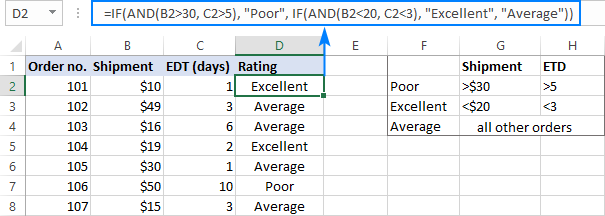More formula examples can be found in Excel nested IF AND statements.

## Case-sensitive IF AND function in Excel

As mentioned in the beginning of this tutorial, Excel IF AND formulas do not distinguish between uppercase and lowercase characters because the AND function is case-insensitive by nature.

If you are working with case-sensitive data and want to evaluate AND conditions taking into account the text case, do each individual logical test inside the EXACT function and nest those functions into your AND statement:

IF(AND(EXACT(cell,"condition1"), EXACT(cell,"condition2")), value_if_true, value_if_false)

For this example, we are going to flag orders of a specific customer (e.g. the company named Cyberspace) with an amount exceeding a certain number, say \$100.

As you can see in the below screenshot, some company names in column B look the same excerpt the characters case, and nevertheless they are different companies, so we have to check the names exactly. The amounts in column C are numbers, and we run a regular "greater than" test for them:

`=IF(AND(EXACT(B2, "Cyberspace"), C2>100), "x", "")`

To make the formula more flexible, you can input the target customer name and amount in two separate cells and refer to those cells. Just remember to lock the cell references with \$ sign (\$G\$1 and \$G\$2 in our case) so they won't change when you copy the formula to other rows:

`=IF(AND(EXACT(B2, \$G\$1), C2>\$G\$2), "x", "")`

Now, you can type any name and amount in the referenced cells, and the formula will flag the corresponding orders in your table: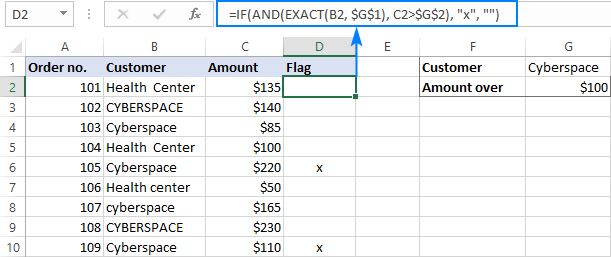## IF OR AND formula in Excel

In Excel IF formulas, you are not limited to using only one logical function. To check various combinations of multiple conditions, you are free to combine the IF, AND, OR and other functions to run the required logical tests. Here is an example of IF AND OR formula that tests a couple of OR conditions within AND. And now, I will show you how you can do two or more AND tests within the OR function.

Supposing, you wish to mark the orders of two customers with an amount greater than a certain number, say \$100.

In the Excel language, our conditions are expressed in this way:

`OR(AND(Customer1, Amount>100), AND(Customer2, Amount>100)`

Assuming the customer names are in column B, amounts in column C, the 2 target names are in G1 and G2, and the target amount is in G3, you use this formula to mark the corresponding orders with "x":

`=IF(OR(AND(B2=\$G\$1, C2>\$G\$3), AND(B2=\$G\$2, C2>\$G\$3)), "x", "")`

The same results can be achieved with a more compact syntax:

`=IF(AND(OR(B2=\$G\$1,B2= \$G\$2), C2>\$G\$3), "x", "")`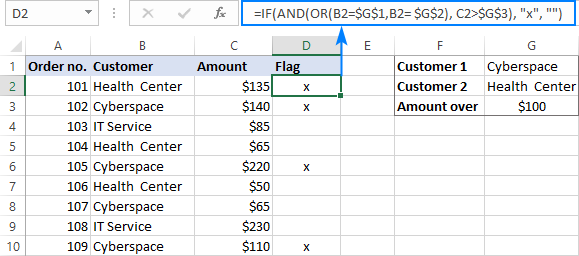Not sure you totally understand the formula's logic? More information can be found in Excel IF with multiple AND/OR conditions.

That's how you use the IF and AND functions together in Excel. To gain more understanding of the formulas, I invite you to download our sample IF AND Excel workbook. Thank you for reading and see you next week!

## You may also be interested in

### 255 comments to "IF AND formula in Excel"

1.Minoe says:

I've been trying to use the IF+AND Function to know if my Focus Data is equal to the Accepted data, Y/N. However, after manually checking, it always comes up with a No despite it being a Yes instead.

My formula is =IF(AND(K2=B2:B186,L2=D2:D186),"Yes","No")

My Focus data are found in Columns K and L. The Accepted Data is found in Columns B and D.

Note:
-I already converted the data to all of these cells to values but it is the same.
-There are duplicate values in the columns except the data found in Column L. Data in Column L are unique.

•Alexander Trifuntov (Ablebits Team) says:

Hi!
The condition K2=B2:B186 returns an array of 185 TRUE/FALSE values. The IF function does not work with arrays. To determine at least one match of K2 with a list of values, you can use SUM(--(K2=B2:B186))

2.Melissa says:

Hello,

I'm trying to return a % based on a range. So for instance. If a discount is between 20%-29%, I want it to return a 3% value. If the discount is between 30% - 39%, I want it to return a 4% value and so on. How do I write this statement? What am I doing wrong?

=IF(AND(J3>20%,J330%,J340%,J3<100%),"5%","")

3.Kevin J Wholley says:

I am stuck on expanding this IF statement. The below IF - AND - OR works fine, but..

I want to expand the about to add another name to the OR check and a different time

for example, I would like A9="Joe" with the F9 check to 8:00 am

I have tried every combination with multiple IF statements but can't seem to expand this check. At some point, I would like to grow this by 8-10 names and 4-5 times.

Any help would be appreciated

•Alexander Trifuntov (Ablebits Team) says:

Hello!
You can find the examples and detailed instructions here: Excel IF statement with multiple AND/OR conditions, nested IF.

=IF(AND(OR(A9="Kevin",A9="Nick"),F9 > = TIME(10,0,0)),"good",IF(AND(A9="Joe",F9 > = TIME(8,0,0)),"good","Bad"))

Instead of nested IF you can use the new Excel IFS function.

4.Marvi says:

Hi, i have a a scenario for programe "Annaul procedure review", with frequencey 1year and 3 years and five years. Plz suggest me formula for that scenario.

5.Wendy Chan says:

Dear Sir,

I have a excel file which created by my superior, I tried to understand how the formula works but in a mist of the logic, please see below the formula:

=IF(AND(ES\$2>=\$M14519,ES\$2<=\$N14519),IF(MONTH(ES\$2)=MONTH(\$M14519),\$K14519/\$Q14519*(ES\$1),\$K14519/\$Q14519*ES\$1),0)

Remark:
ES\$2 = 31 Mar 2021; M14519 = 28 Mar 2021; N14519 = 27 Mar 2022; K14519 = 41600; Q14519 = 365
ES\$1 = 31

Basically this formula created to work out the fee amount by month accordingly to the lump sum amount and the contract start/end period.

I am in a mist of the setup of this part "IF(MONTH(ES\$2)=MONTH(\$M14519)" & what is the relationship of the \$K14519/\$Q14519*(ES\$1),\$K14519/\$Q14519*ES\$1) with the first half of the formula? Why K14519/Q14519*(ES\$1) appeared twice in the formula?

Regards

•Alexander Trifuntov (Ablebits Team) says:

Hello!
It doesn't make any sense that TRUE and FALSE are the same in an IF function. Expression
IF(MONTH(ES\$2)=MONTH(\$M14519),\$K14519/\$Q14519*(ES\$1),\$K14519/\$Q14519*ES\$1)
can be replaced with
\$K14519/\$Q14519*ES\$1

6.Hallie says:

Hello! Can you please assist me on the below?

I have 4 Cells (B4:E4) consisting of dependent drop-down lists where a final solution is to appear in Cell B5. Until the final solution appears, it always indicates "PENDING".

Unfortunately, I have run into a couple of situations where I have received the 8,192 character limit error.

If I have various scenarios that look similar to the below, how could this be written differently to help with my character limit issue? I have attempted practicing with other formulas just on this small set of lines (ex. IF(AND(OR, IF(OR(AND, IFS..), however I cannot make them work but truly I have no experience using those 3 formulas. Forgive me, but you are working with someone who is still learning but definitely tries!

TO SUM UP THE LINES BELOW....

CELL B4 - DIFFERENT IN ALL OF THESE LINES
CELLS C4, D4, E4 - DROP-DOWN'S ALL MATCH IN EACH OF THESE LINES
CELL B5 (THE SOLUTION, OR WHAT IS 'TRUE') - IS THE SAME IN EACH LINE, EXCEPT THE LAST LINE (DUE TO DROP-DOWN B4)

THESE ARE ALL DROP-DOWN POSSIBILITIES WHERE BOTH D4="CLEARLY WRITTEN, COMPLETE" & E4="NO" EXIST TOGETHER. WHAT IS 'TRUE' IN CELL B5 IS THE SAME, EXCEPT WHEN DROP-DOWN B4="DB" IS SELECTED. OTHERWISE, I'D SIMPLY WRITE THIS AS:
=IF(AND(D4="CLEARLY WRITTEN, COMPLETE",E4="NO"),"UPDATE THE SYSTEM TO MATCH THE FORM. DO NOT SEND LETTER.","PENDING")

=IF(AND(B4="EA",C4="YES",D4="CLEARLY WRITTEN, COMPLETE",E4="NO"),"UPDATE THE SYSTEM TO MATCH THE FORM. DO NOT SEND LETTER.",
IF(AND(B4="HP",C4="YES",D4="CLEARLY WRITTEN, COMPLETE",E4="NO"),"UPDATE THE SYSTEM TO MATCH THE FORM. DO NOT SEND LETTER.",
IF(AND(B4="CP",C4="YES",D4="CLEARLY WRITTEN, COMPLETE",E4="NO"),"UPDATE THE SYSTEM TO MATCH THE FORM. DO NOT SEND LETTER.",
IF(AND(B4="WP",C4="YES",D4="CLEARLY WRITTEN, COMPLETE",E4="NO"),"UPDATE THE SYSTEM TO MATCH THE FORM. DO NOT SEND LETTER.",
IF(AND(B4="FN",C4="YES",D4="CLEARLY WRITTEN, COMPLETE",E4="NO"),"UPDATE THE SYSTEM TO MATCH THE FORM. DO NOT SEND LETTER.",
IF(AND(B4="ML",C4="YES",D4="CLEARLY WRITTEN, COMPLETE",E4="NO"),"UPDATE THE SYSTEM TO MATCH THE FORM. DO NOT SEND LETTER.",
IF(AND(B4="SS",C4="YES",D4="CLEARLY WRITTEN, COMPLETE",E4="NO"),"UPDATE THE SYSTEM TO MATCH THE FORM. DO NOT SEND LETTER.",
IF(AND(B4="DB",C4="YES",D4="CLEARLY WRITTEN, COMPLETE",E4="NO"),"UPDATE THE SYSTEM TO MATCH THE FORM. SEND MS1 LETTER.","PENDING"))))))))

Again, these are only a set of lines. If you need more, please just let me know. Here is a list of all drop-down's if preferred.

CELL B4 (CONSISTS OF INFO A FORM IS RECEIVED WITH) =
EA
HP
CP
WP
FN
MI
DB
GE
SS

YES
NO

CELL D4 (THE INFO ON THE FORM IS or CONSISTS OF... dependent drop-downs based on what is selected in cell B4) =
ID PROVIDED
CLEARLY WRITTEN, COMPLETE
WRITTEN, BUT ILLEGIBLE OR INCOMPLETE
REASON PROVIDED INDICATING WHY THEY DID NOT INCLUDE
INVALID IN THE SYSTEM
NOT PROVIDED
BOTH MARKED

E4 (available if C4 drop-down is "YES", asking if the info provided matches what the system currently shows) =
YES
NO

•Hallie says:

Note: Line 6 of formula should have indicated B4="MI" (not B4="ML").... sorry about that :/

•Hallie says:

Me again! DISREGARD! I went back through everything and re-tried the very last example above
** IF AND OR ..... =IF(AND(OR(B2=\$G\$1,B2= \$G\$2), C2>\$G\$3), "x", "") ** ....

It worked on my side as:

=IF(AND(OR(B4="EA",B4="HP",B4="CP",B4="WP",B4="FN",B4="MI",B4="SS"),C4="YES",D4="CLEARLY WRITTEN, COMPLETE",E4="NO"),"UPDATE THE SYSTEM TO MATCH THE FORM. DO NOT SEND LETTER.",
IF(AND(B4="DB",C4="YES",D4="CLEARLY WRITTEN, COMPLETE",E4="NO"),"UPDATE THE SYSTEM TO MATCH THE FORM. SEND MS1 LETTER.","PENDING"))

I am not sure what I did incorrectly when trying it earlier on my side, but thrilled right now! Thank you!

•Alexander Trifuntov (Ablebits Team) says:

Hi!
I didn't quite understand where the error occurred with a large number of characters. But you can reduce the number of characters in the formula if you write a long text in separate cells and make references to these cells in the formula.
=IF(AND(D4=”CLEARLY WRITTEN, COMPLETE”,E4=”NO”),”UPDATE THE SYSTEM TO MATCH THE FORM. DO NOT SEND LETTER.”,”PENDING”)
=IF(AND(D4=”CLEARLY WRITTEN, COMPLETE”,E4=”NO”),M1,”PENDING”)
I hope this will help.

7.Angela Tharp says:

I have formulas that I need to combine together.
D2 = tool size
We have small, med/lg, and critical. So if the mold is above 351 (med/lg) it pulls information from another sheet and put in corresponding row. If under 350 (small), then it put in that row. We have added the "critical" criteria, so I need the formula to look at tool size and look to see if the tool size listed has a "C" after it. If the tool size has a "C" after it, then it needs to go to the critical row, otherwise it needs to go to small or med/l

TOOl # 2
TOOL SIZE 1300C
small 0
med/l 22
critical 22

This didn't work because it still pulled in based on size to the med/l and the critical based on the "C"

•Alexander Trifuntov (Ablebits Team) says:

Hi!
Your task is not completely clear to me. Explain what it means "go to the critical row" and "go to small or med/l".

8.Dahlia says:

Hello there,
Thank you for such insightful site!
I tried following your web but I still don't really get the logic, and when i tried my formula below, some of the case it's good, but some of the case it said FALSE. I think there's something missing in my formula.

Input: Row E is every 25th of the month, no matter what day it is
Wanted Output : I want to create an automatic calendar for every 25th of the month for payroll system.
The condition is, if 25th of the month is a public holiday or weekends (Saturday, Sunday), it should be moved to H-1 (24th) or the nearest working day.

What I get right now:

=IF(COUNTIF(\$H\$22:\$H\$25,E13)>0,IF(WEEKDAY(EDATE(E13,0),12)>5,EDATE(E13,0)-(WEEKDAY(EDATE(E13,0),12)-4),IF(WEEKDAY(EDATE(E13,0),12)5,EDATE(E13,0)-(WEEKDAY(EDATE(E13,0),2)-5),EDATE(E13,0)))))

This is the description of the formula
=IF(COUNTIF(\$H\$22:\$H\$25,E13)>0, [to see If 25th is a public holiday]
IF(WEEKDAY(EDATE(E13,0),12)>5, EDATE(E13,0)-(WEEKDAY(EDATE(E13,0),12)-4),
[if the public holiday falls on Sunday or Monday- 6 or 7, then this is to move the date to nearest weekday - Friday]
IF(WEEKDAY(EDATE(E13,0),12)5,EDATE(E13,0)-(WEEKDAY(EDATE(E13,0),2)-5),[if no, 25th of the month is not a public holiday but it is on weekend Saturday, Sunday, then this is to move the date to nearest weekday - Friday
EDATE(E13,0))))) [if the 25th is a workday]

I hope you understand what I'm trying to say since it is a bit complicated and English is not my first language.
I would very highly appreciate it if you can help me with this!

Many thanks, Dahlia

•Alexander Trifuntov (Ablebits Team) says:

Hello!
If I understand your task correctly, the following formula should work for you:

=IF(WEEKDAY(E13,2) > 5,IF(COUNTIF(\$H\$22:\$H\$25,E13-WEEKDAY(E13,2)+5) > 0,E13-WEEKDAY(E13,2)+4,E13))

•Dahlia says:

I tried the formula but sometimes the result is FALSE

For example, i put the E13 date is Monday, 2nd May 2022 which is a holiday, so it should be Friday, 29 April 2022 but the result written FALSE.

Also, I have a case if the holiday happened at Monday, 2 May 2022 and Friday, 29 April 2022, can you help me to revise the formula?

Thank yiu so much for your help!

•Alexander Trifuntov (Ablebits Team) says:

Hi!
I don't know which days of the week are your holidays. Therefore, if necessary, change the argument of the WEEKDAY function as you need. In this formula, the first day of the week is Monday. Holidays are 6 and 7 days.

=IF(WEEKDAY(E13,2) > 5,IF(COUNTIF(\$H\$22:\$H\$25,E13-WEEKDAY(E13,2)+5) > 0,E13-WEEKDAY(E13,2)+4,E13-WEEKDAY(E13,2)+5),IF(COUNTIF(\$H\$22:\$H\$25,E13) > 0,IF(WEEKDAY(E13,2)=1,E13-3,E13),E13))

•Dahlia says:

Hey! Thank you very much again for replying!
This formula works well, but I'm getting new issue now.

So, if i want to apply the formula to holiday that falls on Tuesday or other weekdays (except monday) then how i should add the formula but different weekday function?

•Dahlia says:

Hi, a little update on the formula so I tried to move here and there, now it kinda work for 3 holidays in a row on the weekdays, but now it can not filter the weekends anymore

here is the formula:

=IF(COUNTIF(\$A\$2:\$A\$18,I26)>0,

IF(WEEKDAY(I26,12)>=5,I26-(WEEKDAY(I26,12)-4),

IF(COUNTIF(\$A\$2:\$A\$18,I26-1)>0,

IF(WEEKDAY(I26-1,12)>=5,I26-1-(WEEKDAY(I26-1,12)-4),

IF(COUNTIF(\$A\$2:\$A\$18,I26-2)>0,

IF(WEEKDAY(I26-2,12)>=5,I26-2-(WEEKDAY(I26-2,12)-4),(I26-3)),(I26-2))),(I26-1))),I26)

I want to fix it but I think it makes the formula even harder, again can you please help me the formula?

•Alexander Trifuntov (Ablebits Team) says:

Hi!
Try to use WORKDAY.INTL function:

=WORKDAY.INTL(E13+1,-1,1,H22:H25)

This will help you find the nearest working day, taking into account holidays and weekends.
Hope this is what you need.

•Alexander Trifuntov (Ablebits Team) says:

Hi!
You can set which day of the week is a weekend using the second argument of the WEEKDAY function.

9.Shannon Peters says:

Hello, I have multiple conditions for calculating faculty workload and can't seem to get my formula right. The original formula was:

However I need to check some conditions before performing the operation above.
-If the total workload credits are >= 24
-And the total contact hours are >= 48

Is this possible?

10.Robert says:

I would like to use the If(And) formula shown in this page but looking at distinct numbers in a large excel like user id's, for example. How could I use this formula to search many rows of data for a specific column?

I was thinking vlookup with the IF(And) within it but I was unable to get the syntax correct.

•Alexander Trifuntov (Ablebits Team) says:

Hi!
Sorry, it's not quite clear what you are trying to achieve.

11.Georgina Richardson says:

Hi there,
Thank you for such a valuable site!
Question: I have a check out system for items where I need the item to show status in column C:
-Blank if there is no date entered as due
-"Not due" if there is a date that does not exceed today's date
-"Overdue" if there is a date past today's date
-"Returned" if there is a date entered into the Returned cell.
Column C is Status, D is Item, E is Name, F is Notes, G is Due Back Date, H is Returned Date
The formula I have so far that works to address the first 3 criteria using line 8 as my example:
=IF(ISBLANK(G8),"",IF(G8<TODAY(),"Overdue","Not due"))
but I cannot figure out how to get the final criteria to supersede the other criteria if a return date is present in H8 with a result of "Returned".
If you can help with this I would appreciate it very much!
Many thanks - Georgina

•Georgina Richardson says:

Totally worked thank you SO much!! I really appreciate the help!

•Georgina Richardson says:

As fate would have it - I actually saw the formula working and now it is no longer working....
Here is what I have on line 8:
=(ISNUMBER(H8),"Returned",IF(ISBLANK(G8),"", IF(G8<TODAY(),"Overdue","Not due")))
Initially this exact formula showed a value of "Returned", when a return date was entered into H8. After going back to it and entered more data it only showed "Overdue" as the value even though a date (correct format) was entered into the Returned Date field, now I am receiving nothing but a "There's a problem with this formula" prompt. Please help again...

•Alexander Trifuntov (Ablebits Team) says:

Hello!
You didn't exactly copy the formula.

=IF(ISNUMBER(H8),"Returned",IF(ISBLANK(G8),"",IF(G8 < TODAY(),"Overdue","Not due")))

•Georgina Richardson says:

Well that's embarrassing - my apologies for wasting your time in responding as I have been using the full formula and it is not working
This is a direct paste from the first line (row 2):
=IF(ISNUMBER(H2),"Returned",IF(ISBLANK(G2),"",IF(G2 < TODAY(),"Overdue","Not due")))

Am I missing something else?
Georgina

•Alexander Trifuntov (Ablebits Team) says:

Hi!
I'm not sure I understand your conditions, but try this formula -

=IF(ISBLANK(G8),"",IF(ISNUMBER(H8),"Returned",IF(G8 > TODAY(),"Overdue","Not due")))

•Georgina Richardson says:

With 2 minor tweaks of your formula corrections it is now working. Here's what ended up working:
=IF(ISBLANK(G2),"",IF(ISTEXT(H2),"Returned",IF(G2 > TODAY(),"Not due","Overdue")))

The "ISNUMBER" would not accept a date as the data so I changed it to "ISTEXT" and switched Overdue and Not due and all working now.

If it wasn't for your kindness in giving alternate solutions I would still be experiencing such frustration.

Alexander - Thank you thank you thank you!!

•Alexander Trifuntov (Ablebits Team) says:

Hi!
ISNUMBER doesn't work because your date is written as text. This is not normal, but I cannot know about it. For a normal date, ISNUMBER works, since a date is a number.

•Georgina Richardson says:

You have been extremely helpful - cannot thank you enough!

•Michelle says:

Good day from South Africa. I am struggling with a formula containing AND and OR.
Question:
All books with cost prices between \$50 and \$80 (both prices included) on Accounting (Acc) and Tax supplied by the publisher Butterworths. Cost price is in column F, Type of book is in Column D and the publisher is in column E.
My formula:
=and(F4>=50,F4<=80),OR(D4="Tax",D4="Acc"),AND(E4="Butterworths")

Why is my formula not working?
Thank you

•Michelle says:

On the same topic I also have the following question where my formula is not working:

Extract a list of all Afrikaans, English and Japanese (Column I) books and DVD's (Column G) that were issued since 2000 (Column D), the year 2000 excluded.

My formula:
=OR(I2="English",I2="Afrikaans,I2="Japanese),OR(G2="Book",G2="DVD"),AND(D2>2000)

Please help me - I would be very grateful. I find putting the formula in three different line using the ALT + ENTER option, splits them so that I can understand them, but as soon as I need to string them together, I somehow fail.

Thank you and kind regards

12.Nishantha says:

can help me to work my formula. I need generate PASS Or fail with sample size and major and minor damage.
=IF(J3=0,"PASS","FAIL"),IF(I3="13",IF(K3<2,"PASS",IF(L3<3,"PASS","FAIL")),IF(I3="20",IF(K3<3,"PASS",IF(L3<4,"PASS","FAIL")),IF(I3="32",IF(K3<4,"PASS",IF(L3<6,"PASS","FAIL")),IF(I3="50",IF(K3<6,"PASS",IF(L3<8,"PASS","FAIL")),IF(I3="80",IF(K3<8,"PASS",IF(L3<11,"PASS","FAIL")),IF(I3="125",IF(K3<11,"PASS",IF(L3<15,"PASS","FAIL")),IF(I3="200",IF(K3<15,"PASS",IF(L3<22,"PASS","FAIL")),IF(I3="300",IF(K3<17,"PASS",IF(L3<24,"PASS","FAIL"))))))))))

13.Cyril says:

Hello

I have a list of students from countries all over the world and I would like to complete a column which says which continent they are from.

For example if a students comes from Spain , I want Europe to be written in the continent column and find a formula that can do that.
I don't know if it's possible though.
I would like to have all the continents ( Europe, Amercia , Asia ...) and every country on our list to be enclosed in one specific continent. We have almost all countries in the world, it's a lot of names.

Something like if (student column A) ( column B spain , France, italy , germany (and so on) , " Europe" " America" "Asia".. )

I went through all the examples mentionned and I tried many times different formulas but I can get my head around it. Help would be highly appreciated

Many thanks
Cyil

•Cyril says:

I had a look at this function but it's not what I am looking for because from the list of students coming from all over the world I won't be able to differenciate the countries and have a specific country aattributed to matching continents

I had another idead, I have listed European countries under the number 1 , Asian countries under th number 2 and so on ..
I am now looking to have a function to say in colum A (the countries from the students list I want to identify as continents) in the column the following (B1:B5) is Europe , (B16:B50) is Asia ...) so if B16 then it's Asia , if it's B4 then it's Europe

Sorry I hope I made myself clear

•Alexander Trifuntov (Ablebits Team) says:

Hello!
Unfortunately, I can't understand why the VLOOKUP function is not suitable for you and what you want to do.

14.Srinivasan says:

Hi, I need an help in the IF formula in number and text combined. I will post the test can resolve this for or suggest the what can I do for this.

A B
CODE CATEGORY
1600 A Team
1601 A Team
1602 A Team
1603 A Team
1604 B Team
1605 B Team
1606 B Team

If I enter the any code number in A2 cell and the B2 auto pick the correct team. this is what i want can you please give me the solution for this.

I have 100 combination like this and to complete the task my self only.

•Alexander Trifuntov (Ablebits Team) says:

Hello!
If I understand the problem correctly, you can find the command that matches the code using the VLOOKUP function. You can find examples and detailed instructions in this article.

•Srinivasan says:

Thank you for the help Mr. Alex

15.Monica C. says:

Hi! I have built a formula that calculates when we need to send out an order (20 days after receiving previous order), based on how many months were ordered. I built in an IF function that hides numbers if there isn't any data in the formula. Now I want to add into the formula that stops calculating a ship date if there are no more paid orders. I have another column that has how many months were purchased. How do I integrate an AND function into this that would only calculate if months>2? I cannot get the formula to compute.

•Alexander Trifuntov (Ablebits Team) says:

Hi!
If I understand your task correctly, the following formula should work for you:

•Monica C says:

Hi!

Thanks so much for the response. For some reason, it isn't. It is still calculating, even when the number of months is 2. The formula makes sense to me as well.

16.Gab says:

Hey, i am looking for formula that can calculate at different amounts. For example. I have 40 apples, but the first 10 apples are at \$2, the other 11 to 30 apples @ \$2.50 and any apples over 40 @ \$3.00. Then get the summation of all this in one cell.

can someone help.

thanks

17.Michael Hanson says:

Hi I am trying to work out a formula to update on cell based on the conditions of 2 cells.
If Cell 1 = 2 and Cell 2 = Yes then cell 3 should = No. Hope that is a clear expalanation

•Alexander Trifuntov (Ablebits Team) says:

Hi!

18.natasha says:

Previously posted incorrectly.

I am trying to get formula to return either 19/20 20/21 or 21/22 depending on when the date falls.

However the formula does not seem to be working.

'=IF(AND(x>=01.04.2019,x=01.04.2020,x=01.04.2021,x<=31.03.2022),'21/22',"-"

•Alexander Trifuntov (Ablebits Team) says:

Hi!

19.natasha says:

I cannot understand why my formula is not working.
=IF(AND("x">="01.04.2019","x"="01.04.2020","x"="01.04.2021","x"<="31.03.2022"),"21/22","--"

•Alexander Trifuntov (Ablebits Team) says:

Hello!
You need a parenthesis at the end of your formula. Then it will work properly if it matches your data.

=IF(AND("x">="01.04.2019","x"="01.04.2020","x"="01.04.2021","x"<="31.03.2022"),"21/22","--")

20.Belinda Young says:

I am working to get a formula that calculates if (D6 says "Yes" and C6 says "Pay Run" ,B6*26) or if (D6 Says "Yes" and C6 says "Month", B6*12) or if (D6 says "Yes" and C6 says "Year", B6*1) or if D6 says "No" return 0.00.

I have tried =IF(AND(D6="Yes",C6="Pay run"),B6*26,IF(OR(D6="Yes",C6="Month"),B6*12,IF(OR(D6="Yes",C6="Year"),B6*1,"0"))) and this works for D6 saying "Yes" and C6 saying "Pay Run" and D6 saying "Yes" and C6 saying "Month" but doesn't work for D6 saying "Yes" and C6 saying "Year" or if D6 says "No"

Can you help?

•Alexander Trifuntov (Ablebits Team) says:

Hi!
Replace OR with AND in the formula

=IF(AND(D6=”Yes”,C6=”Pay run”),B6*26,IF(AND(D6=”Yes”,C6=”Month”),B6*12,IF(AND(D6=”Yes”,C6=”Year”),B6*1,”0″)))

•Belinda Young says:

Thank you for your quick reply! This works perfectly - thank you!

21.Marsh says:

I'm trying to return a date in another worksheet if S3 is showing an N/A. I'm using this formula that is working but I need to go a step further. I'm looking to show blank/nothing in the cell if S3 doesn't equal N/A, what do i need to add to make that happen?

=IFNA(S3,VLOOKUP(@N:N,Completed_Ocean[[Equipment '#]:[Date and Time '@ Consolidator]],26,FALSE))

Thanks!

•Alexander Trifuntov (Ablebits Team) says:

Hello!
Use an IF formula with condition an ISNA function

=IF(ISNA(S3),VLOOKUP(@N:N,Completed_Ocean[[Equipment ‘#]:[Date and Time ‘@ Consolidator]],26,FALSE),"")

Hope this is what you need.

•Marsh says:

This worked, thanks for the help and all the insight the site provides!!

22.Steph Mannix says:

Hi

i need help doing something very simple - i need a certain cell to say 0 if the cell previous says CLOSED.

What formula do i use?

thanks,

•Alexander Trifuntov (Ablebits Team) says:

Hi,
Try this formula:

=IF(A1="CLOSED",0,"")

23.Adelle Gardner says:

I am trying to do a compensation file based on full-time and part-time FTE to come back with -\$500 if they do not meet a certain number of meetings. I can't get the below formula to work, but the work separately but I need to combine them. Any/all help with this matter is greatly appreciatied

=IF((AND(\$F:\$F>=0.51,\$R:\$R<3)),-500,0),OR(IF((AND(\$F:\$F<=0.5,\$R:\$R<1.5)),-500,)

Fulltime fte 3 or more meetings or -500
parttime fte l.5 or more meetins or -500

•Alexander Trifuntov (Ablebits Team) says:

Hi!
Based on your description, it is hard to completely understand your task. However, I’ll try to guess and offer you the following formula:

=IF((AND(\$F:\$F>=0.51,\$R:\$R<3)),-500,IF((AND(\$F:\$F<=0.5,\$R:\$R<1.5)),-500,0))

24.Abraham Crowe says:

I am Trying to =if(and(E11="Gram",G11>=1.08,G11=3.7,G11<=4),"Pass","Fail"))) then I will add more depending on packaging requirements. How do I Create a single "If/and formula" all based on what Product is entered into Column E to show a pass fail in column I based on the weights entered into column G?

25.Cindy Wei says:

Hello! I am trying to set up a code with two conditions and three options within each condition. The value I would like to generate in E depends on BOTH columns C and D.

I would like to do the following if the value in D is =30,"3",
IF(C1>=25,"2",
IF(C1>=20,"1","0"))) /=70:
=IF(C1>=30,"3",
IF(C1>=25,"2",
IF(C1>=22,"1","0"))) /=20 and D/=22 and D>/=70.

I understand how to do these arguments separately (as written above), but I would like to combine the arguments into a single code, where, depending on the value in D, different conditions for C are applied. Not sure how to apply 'AND' arguments in this scenario. Thank you for your help!

•Alexander Trifuntov (Ablebits Team) says:

Hello!
According to your formula, I cannot understand all the conditions. What is D is = 30, ”3 ″ or D / = 22 and D> / = 70?
At the same time, the article above has all the necessary information to write the formula

26.RoHer says:

Hi, Thank you for the great content. I have a question related to the the following formula I have created:

=OR(AND(\$BB63>=P\$7,\$BB63

•Alexander Trifuntov (Ablebits Team) says:

Hi!
I am not sure I fully understand what you mean. Part of the formula is missing.
Please describe your problem in more detail. Write an example of the source data and the result you want to get.

27.Celea Naluburwa says:

Hello Sir,

How do you formulate a scenario like this "if studentA is taking a maths subject this semester as per this class registration list, then they should pay \$1000.
I have list of students pursuing different subjects this semester, but each subject is charged a different rate.

Thank you!

•Alexander Trifuntov (Ablebits Team) says:

Hello!
You didn't say what your registration lists look like. You can use the VLOOKUP or INDEX + MATCH function to search the list. You can find the examples and detailed instructions here: Advanced VLOOKUP in Excel with formula examples.
I hope this will help, otherwise please do not hesitate to contact me anytime.

28.Wendy Roberts says:

I am trying to find a formula to calculate a commission value.

Here is the table:

Goal Attainment Commission Value
0 - 15% \$- 0.00
16% - 30% \$10.00
31% - 40% \$12.00
41% - 50% \$15.00
51%+ \$20.00

The formula I came up with is:

=IFS(J4<16,”0”,J4<31,”10”,J4<41,”12”,J450,"20")

This keeps resulting in #NAME?

I am using MS Excel for Mac version 16.54

•Wendy Roberts says:

sorry, type O in above table. this is what I am using

=IFS(J4<16,”0”,J4<31,”10”,J4<41,”12”,J450,"20")

•Alexander Trifuntov (Ablebits Team) says:

Hello!
The IFS function is available starting with Excel 2019.

29.Erik says:

Is there a way to have the "value_if_true" be the contend of a cell that is not always the same? (i.e. a name generated by a sign up list)

I tried this: =IF(AND(\$A\$3="Ms.Atchison",\$B\$3=G\$1),\$C\$3," ")

The false value is just leaving the cell blank. In C3 is the name of the person that signed up with Ms. Atchison. \$B3\$3=G\$1 is the time of the confernce transposed.

•Alexander Trifuntov (Ablebits Team) says:

Hi!
Sorry, I don't understand what you want to do.

•Erik says:

11/11/21 3:30 PM 3:40 PM 3:50 PM
Conference Name Appt Time Last Name First Name
Ms. Atchison 6:30 PM Kim-Gorup Lia
Mrs. Blanchard 3:30 PM Turner Levi
Mrs. Blanchard 3:40 PM Rectenwald June
Mrs. Blanchard 3:50 PM Robinson Rayna

The report I was able to download has all of the conferences listed as seen on the left: teacher name (column A), Appt. Time (column B), Student last name (column C). I would like to transpose this information so the times are in columns start in column G through column AD (10 minute intervals). So when the time in column B is equal to time in column G through AD AND the teacher name is in column A, the last name listed in column C will show up in the cell under the matching time. I would then have the teacher name listed in column F with the last names under the times going across the row.

Hopefully that makes more sense.

•Alexander Trifuntov (Ablebits Team) says:

Hello!
Unfortunately, your question is not clear. What do you want to write in the cell under the time - a name from A1 or from C1?

•Erik says:

I am wanting to have the name in column C1, which in this case would be Kim- Gorup.

•Alexander Trifuntov (Ablebits Team) says:

Hi!
Your formula prints the name from cell C3 in one of the cells F3:AD3 versus the time in cells F1:AD1. Isn't that what you want?

•Erik says:

Yes, it is what I want, however, if I change the teacher's name from "Mrs. Atchison" to another teacher, that shows up in column A more than once, it will only fill the first name in Column C even if all the other criteria match.

For example: Blanchard is in the next 13 cells in column A (A4-A16) with various times in Column B and different names in Column C.

If I adjust the formula, removing \$ before the cell number, before \$A3 and \$B3, it will pick up the first conference for Blanchare at 3:30 (time in B4), but it will not copy across the row and pick up the conferences that show up in lines A5-A16.

30.Rohan says:

Good Day sir,

What formula could I use to write the following in excel:

if =26 but =51 but =76 but <=100 Print 10%

31.Joe Stelma says:

I am looking for a formula that does the following for an answer in cell J2:
If cell B2=300 then =SUM(D2)*0.03
If cell B2=400 then =SUM(D2)*0.04
If cell B2=500 then =SUM(D2)*0.05

In other words, cell J2 will calculate IF cell B2 is equal to 300, 400 or 500...then the result will calculate the amount in cell D2 and multiply it by 0.03,0.04 or 0.05.

I thought this was simple enough...but can't quite get it right. Any assistance would be greatly appreciated!

•Alexander Trifuntov (Ablebits Team) says:

Hello!
You can use this formula:

=IF(B2=300,D2*0.03,IF(B2=400,D2*0.04,IF(B2=500,D2*0.05,"")))

or

=IFS(B2=300,D2*0.03,B2=400,D2*0.04,B2=500,D2*0.05)